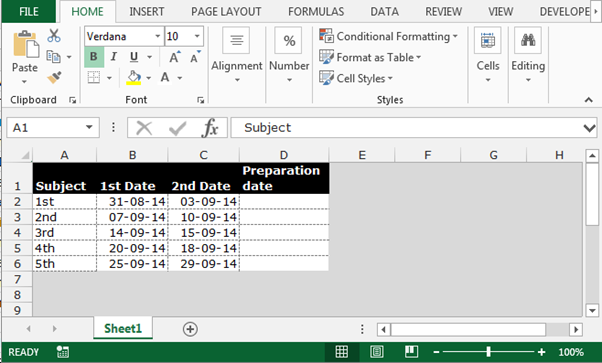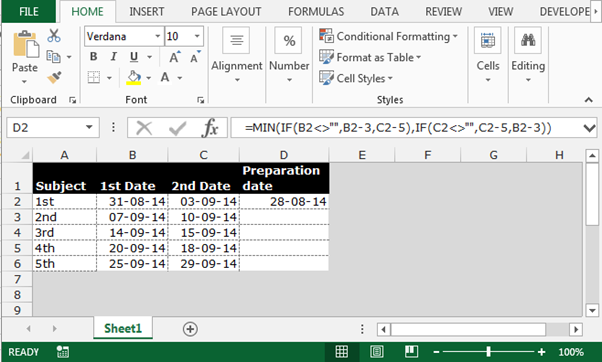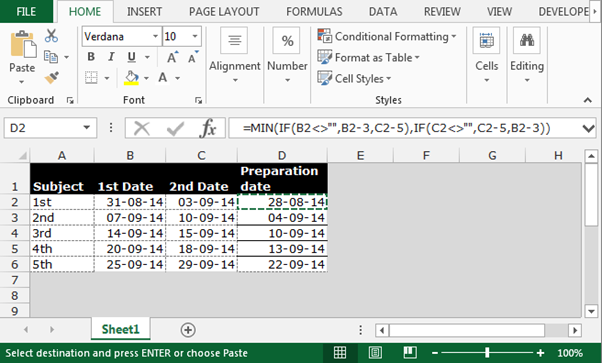Calculating a Required Date According to Two Criteria in Microsoft Excel

To calculate required date according to two criteria, we will use MIN function along with IF function in Microsoft Excel.

• MIN function search for minimum value within a range
• IF function checks define condition is meeting or not

Let’s take an example,

Columns B & C contain test dates and paper deadlines for each of the subjects listed in column A.

A test requires 3 days of preparation and a paper requires 5 days.For each subject, we want to calculate the earliest date required to start preparing for either a test or a paper.

• Write the formula in cell D2
• =MIN(IF(B2<>"",B2-3,C2-5),IF(C2<>"",C2-5,B2-3))
• Press Enter
• The function will calculate the required date• To return the all preparation date copy, use the same formula by pressing Ctrl+C and paste in the range D3:D6 by pressing  Ctrl+VIn this way, we can calculate the require according two criteria in Microsoft Excel.